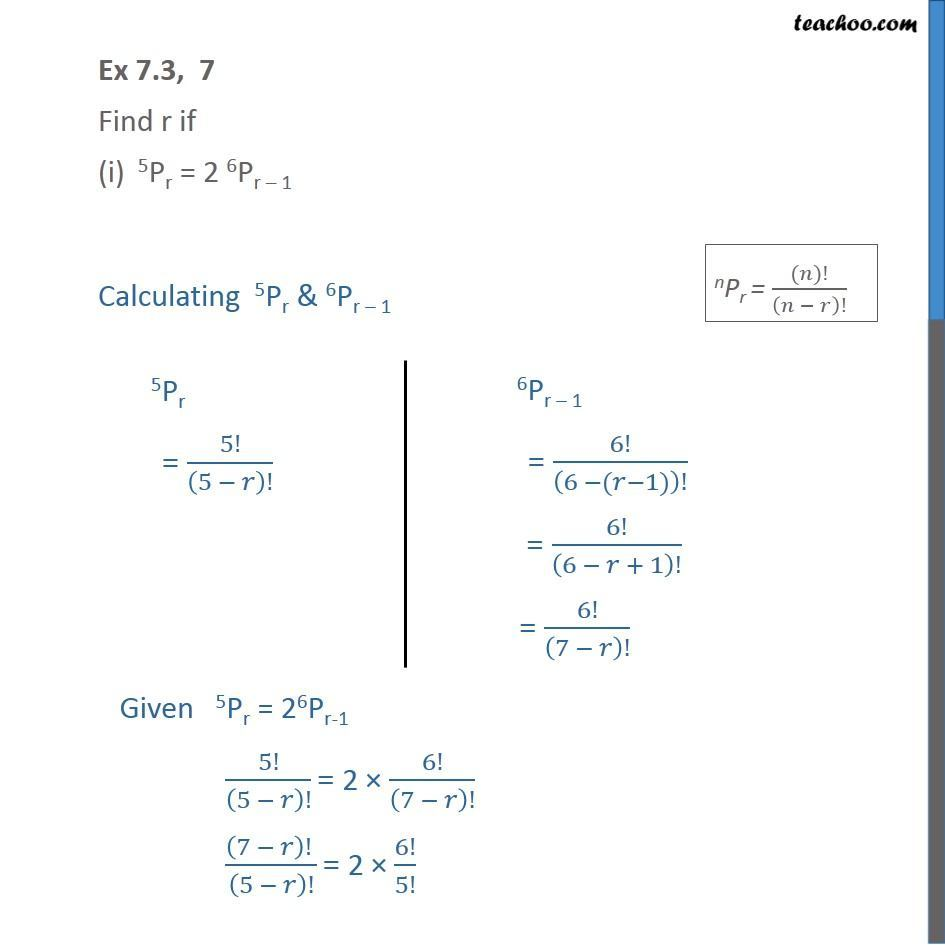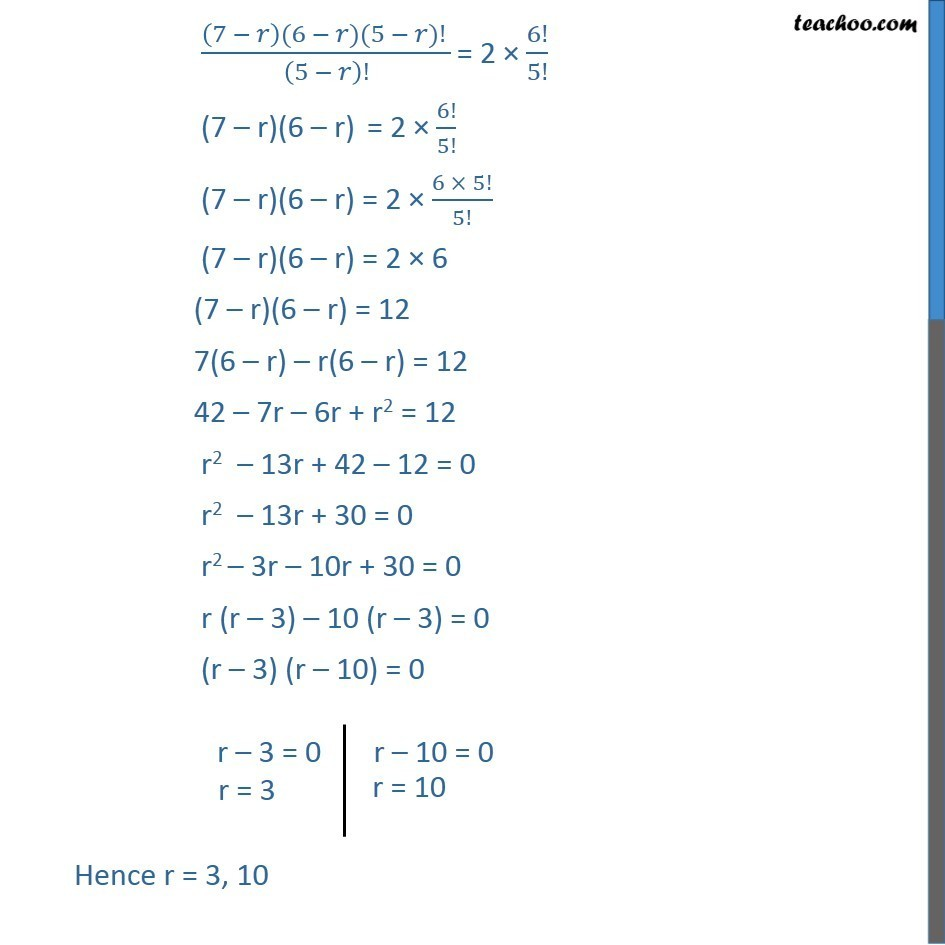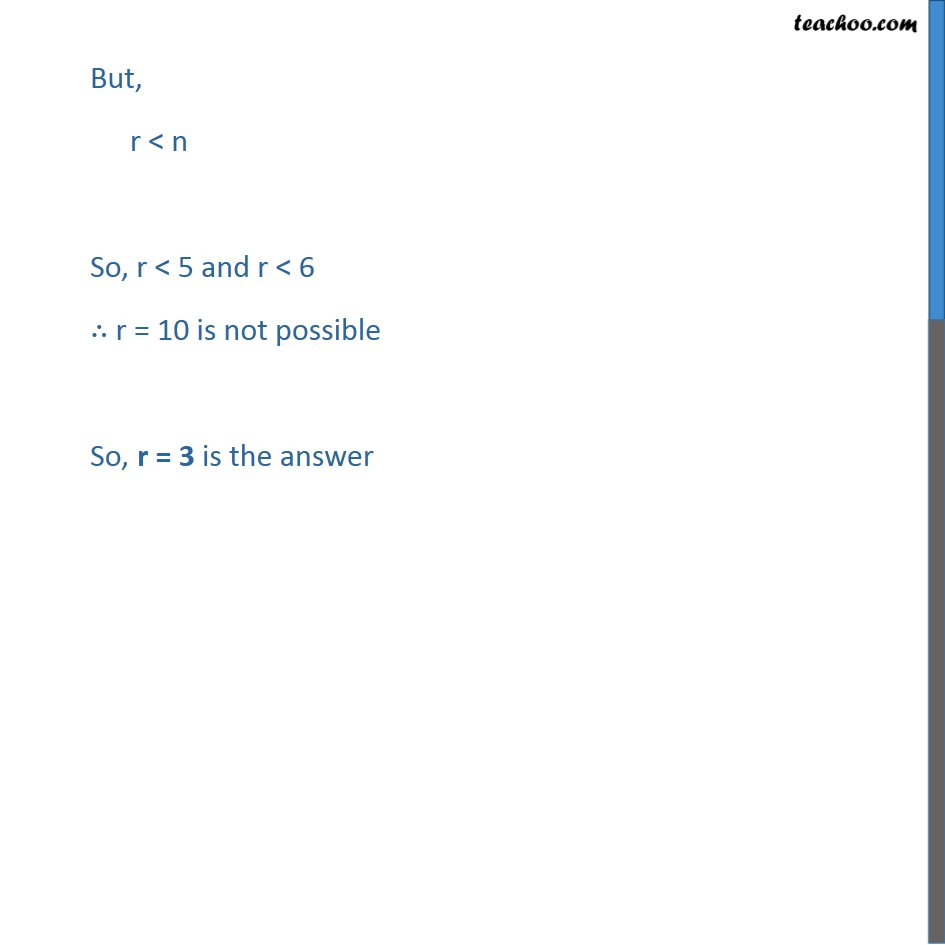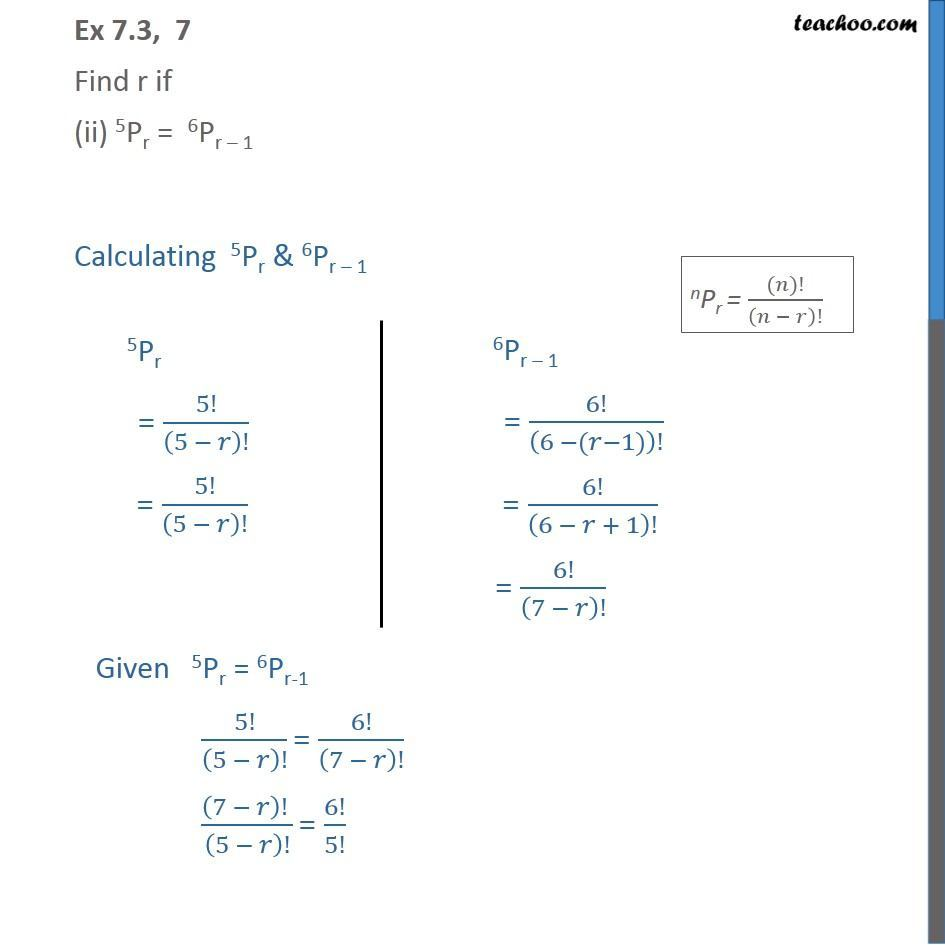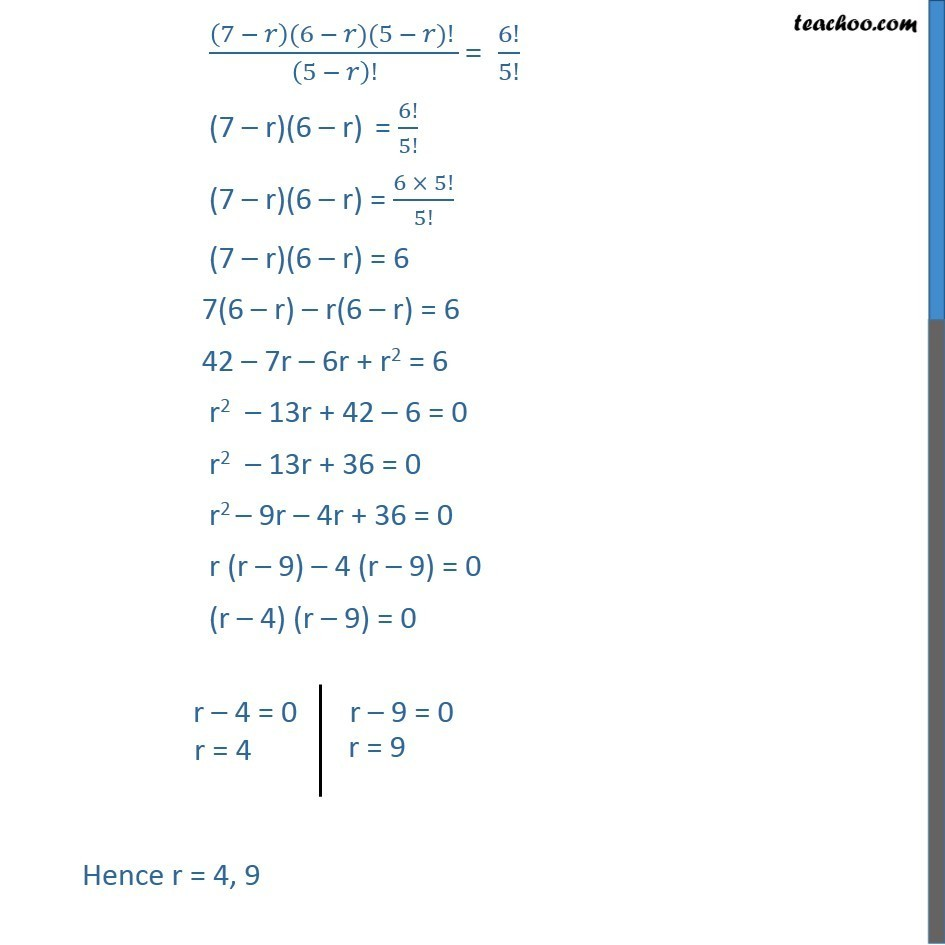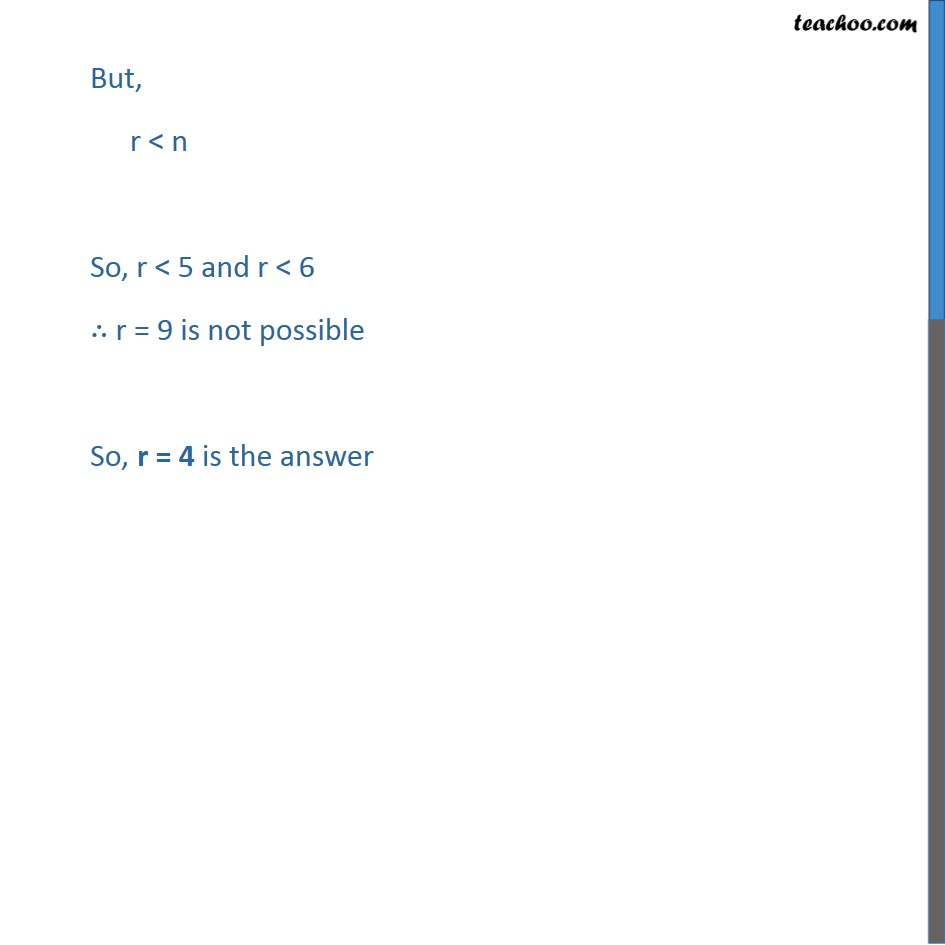1. Chapter 7 Class 11 Permutations and Combinations
2. Serial order wise
3. Ex 7.3

Transcript

Ex 7.3, 7 Find r if 5Pr = 2 6Pr 1 Calculating 5Pr & 6Pr 1 Given 5Pr = 26Pr-1 5!/(5 )! = 2 6!/(7 )! ((7 )! )/(5 )! = 2 6!/5! ((7 )(6 )(5 )! )/(5 )! = 2 6!/5! (7 r)(6 r) = 2 6!/5! (7 r)(6 r) = 2 (6 5!)/5! (7 r)(6 r) = 2 6 (7 r)(6 r) = 12 7(6 r) r(6 r) = 12 42 7r 6r + r2 = 12 r2 13r + 42 12 = 0 r2 13r + 30 = 0 r2 3r 10r + 30 = 0 r (r 3) 10 (r 3) = 0 (r 3) (r 10) = 0 Hence r = 3, 10 Ex 7.3, 7 Find r if (ii) 5Pr = 6Pr 1 Calculating 5Pr & 6Pr 1 Given 5Pr = 6Pr-1 5!/(5 )! = 6!/(7 )! ((7 )! )/(5 )! = 6!/5! ((7 )(6 )(5 )! )/(5 )! = 6!/5! (7 r)(6 r) = 6!/5! (7 r)(6 r) = (6 5!)/5! (7 r)(6 r) = 6 7(6 r) r(6 r) = 6 42 7r 6r + r2 = 6 r2 13r + 42 6 = 0 r2 13r + 36 = 0 r2 9r 4r + 36 = 0 r (r 9) 4 (r 9) = 0 (r 4) (r 9) = 0 Hence r = 4, 9 But, r < n So, r < 5 and r < 6 ∴ r = 9 is not possible So, r = 4 is the answer

Ex 7.3